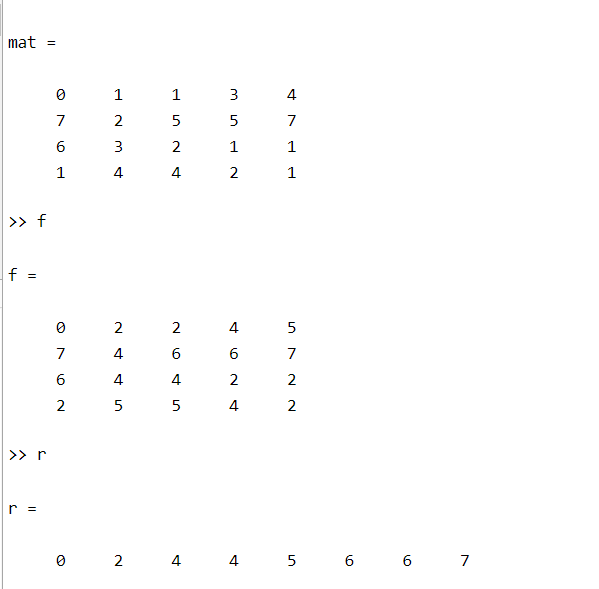# How to Solve Histogram Equalization Numerical Problem in MATLAB?

Histogram Equalization is a mathematical technique to widen the dynamic range of the histogram. Sometimes the histogram is spanned over a short range, by equalization the span of the histogram is widened. In digital image processing, the contrast of an image is enhanced using this very technique.

### Use of Histogram Equalization:

It is used to increase the spread of the histogram. If the histogram represents the digital image, then by spreading the intensity values over a large dynamic range we can improve the contrast of the image.

### Algorithm:

• Find the frequency of each value represented on the horizontal axis of the histogram i.e. intensity in the case of an image.
• Calculate the probability density function for each intensity value.
• After finding the PDF, calculate the cumulative density function for each intensity’s frequency.
• The CDF value is in the range 0-1, so we multiply all CDF values by the largest value of intensity i.e. 255.
• Round off the final values to integer values.

Example:

## Matlab

 `% MATLAB code for Histogram equalisation ` `% function to return resultant ` `% matrix and summary ` `function` `[f,r]=HistEq(k,max) ` `Freq=zeros(1,max); ` `[x,y]=size(k); ` ` `  `% Calculate frequency of each ` `% intensity value. ` `for` `i=1:x ` `    ``for` `j=1:y ` `        ``Freq(k(i,j)+1)=Freq(k(i,j)+1)+1; ` `    ``end` `end` ` `  `% Calculate PDF for each intensity value. ` `PDF=zeros(1,max); ` `Total=x*y; ` `for` `i=1:max ` `    ``PDF(i)=Freq(i)/Total; ` `end` ` `  `% Calculate the CDF for each intensity value. ` `CDF=zeros(1,max); ` `CDF(1)=PDF(1); ` `for` `i=2:max ` `    ``CDF(i)=CDF(i-1)+PDF(i); ` `end` ` `  `% Multiply by Maximum intensity value ` `% and round off the result. ` `Result=zeros(1,max); ` `for` `i=1:max ` `    ``Result(i)=uint8(CDF(i)*(max-1)); ` `end` ` `  `% Compute the Equalized image/matrix. ` `mat=zeros(size(k)); ` `for` `i=1:x ` `    ``for` `j=1:y ` `        ``mat(i,j)=Result(k(i,j)+1); ` `    ``end` `end` `   `  `f=mat; ` `r=Result; ` `end` ` `  `% Utility code here. ` `k=[0, 1, 1, 3, 4;  ` `   ``7, 2, 5, 5, 7;  ` `   ``6, 3, 2, 1, 1;  ` `   ``1, 4, 4, 2, 1]; ` `    `  `[new_matrix, summary]=HistEq(k,8); `

Output:A 3-bit image of size 4×5 is shown below. Compute the histogram equalized image.

Steps:

• Find the range of intensity values.
• Find the frequency of each intensity value.
• Calculate the probability density function for each frequency.
• Calculate the cumulative density function for each frequency.
• Multiply CDF with the highest intensity value possible.
• Round off the values obtained in step-5.
```Overview of calculation:
Range of intensity values = [0, 1, 2, 3, 4, 5, 6, 7]
Frequency of values = [1,  6, 3, 2, 3, 2, 1, 2]
total = 20 = 4*5
Calculate PDF = frequency of each intensity/Total sum of
all frequencies, for each i value of intensity
Calculate CDF =cumulative frequency of each intensity
value = sum of all PDF value (<=i)
Multiply CDF with 7.
Round off the final value of intensity.```

The tabular form of the calculation is given here:

```Interpretation:
The pixel intensity in the image has modified.
0 intensity is replaced by 0.
1 intensity is replaced by 2.
2 intensity is replaced by 4.
3 intensity is replaced by 4.
4 intensity is replaced by 5.
5 intensity is replaced by 6.
6 intensity is replaced by 6.
7 intensity is replaced by 7.```

Output: The new image is as follow:

Whether you're preparing for your first job interview or aiming to upskill in this ever-evolving tech landscape, GeeksforGeeks Courses are your key to success. We provide top-quality content at affordable prices, all geared towards accelerating your growth in a time-bound manner. Join the millions we've already empowered, and we're here to do the same for you. Don't miss out - check it out now!

Previous
Next